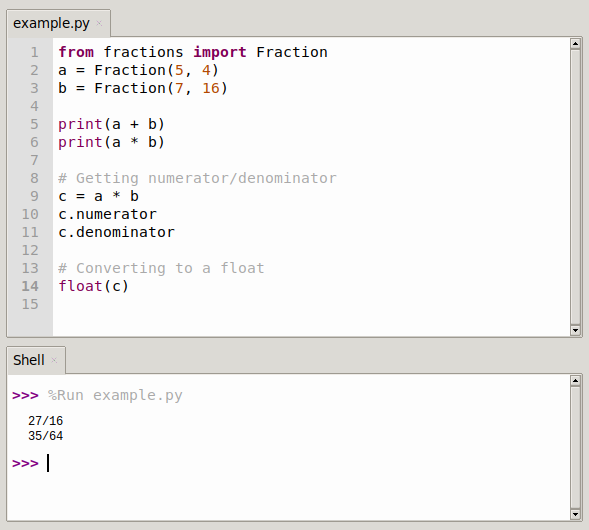# Python fractions module

The Fraction class implements numerical operations with rational numbers.
Basic example:

``````>>> from fractions import Fraction
>>> a = Fraction(5, 4)
>>> b = Fraction(7, 16)
>>> print(a + b)
27/16
>>> print(a * b)
35/64
>>> # Getting numerator/denominator
>>> c = a * b
>>> c.numerator
35
>>> c.denominator
64
>>> # Converting to a float
>>> float(c)
0.546875``````

## Creation of a Fraction instance

Similar to the decimal module, new values can be created in a variety of ways. An easy way to do this is by creating separate numerator and denominator values.

``````import fractions
for n, d in [(1, 2), (2, 4), (3, 6)]:
f = fractions.Fraction(n, d)
print('{}/{} = {}'.format(n, d, f))``````

Keep the lowest common denominator when calculating new values.
This outputs:

``````1/2 = 1/2
2/4 = 1/2
3/6 = 1/2``````

Another way to create a Fraction is to use the / string representation.

``````import fractions
for s in ['1/2', '2/4', '3/6']:
f = fractions.Fraction(s)
print('{} = {}'.format(s, f))``````

Parse this string to find the numerator and denominator values.
Same output:

``````1/2 = 1/2
2/4 = 1/2
3/6 = 1/2``````

Strings can also use the more common decimal or floating-point notation, which is a series of numbers separated by a decimal point. All strings that can be parsed by float() and do not represent NaN or infinity values are supported.

``````import fractions
for s in ['0.5', '1.5', '2.0', '5e-1']:
f = fractions.Fraction(s)
print('{0:>4} = {1}'.format(s, f))``````

The numerator and denominator values expressed as floating point values are automatically calculated.

``````0.5 = 1/2
1.5 = 3/2
2.0 = 2
5e-1 = 1/2
>>> ``````Fraction instances can also be created directly from other representations of rational numbers (such as float or decimal).

``````import fractions
for v in [0.1, 0.5, 1.5, 2.0]:
print('{} = {}'.format(v, fractions.Fraction(v)))``````

Output:

``````0.1 = 3602879701896397/36028797018963968
0.5 = 1/2
1.5 = 3/2
2.0 = 2``````

Floating point values that cannot be accurately represented may yield unexpected results.

Using the Decimal representation of the value will give the desired result.

``````import decimal
import fractions
values = [
decimal.Decimal('0.1'),
decimal.Decimal('0.5'),
decimal.Decimal('1.5'),
decimal.Decimal('2.0'),
for v in values:
print('{} = {}'.format(v, fractions.Fraction(v)))``````

Decimal’s internal implementation does not have the precision error of a standard floating-point representation.

## Arithmetic operations

Once the fraction is instantiated, it is ready for use in mathematical expressions.

``````import fractions
f1 = fractions.Fraction(1, 2)
f2 = fractions.Fraction(3, 4)
print('{} + {} = {}'.format(f1, f2, f1 + f2))
print('{} - {} = {}'.format(f1, f2, f1 - f2))
print('{} * {} = {}'.format(f1, f2, f1 * f2))
print('{} / {} = {}'.format(f1, f2, f1 / f2))``````

Fractional operations support all standard operators.
output:

``````1/2 + 3/4 = 5/4
1/2 - 3/4 = -1/4
1/2 * 3/4 = 3/8
1/2 / 3/4 = 2/3``````

## Approximate value

Fraction has the useful property of being able to convert a floating point into an approximate plausible value.

``````import fractions
import math
print('PI =', math.pi)
f_pi = fractions.Fraction(str(math.pi))
print('No limit =', f_pi)
for i in [1, 6, 11, 60, 70, 90, 100]:
limited = f_pi.limit_denominator(i)
print('{0:8} = {1}'.format(i, limited))``````

The value of this fraction can be controlled by limiting the denominator size.